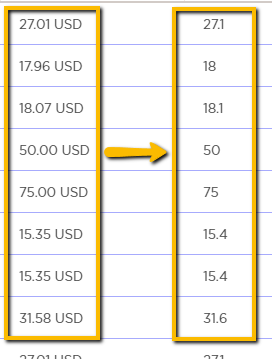# How can I apply mathematical actions? (EM)

#### Before you begin

Make sure the column type is set to Number (click this link to learn how).

#### Math

Create mathematical actions such as addition, subtraction, multiplication, or division with numerical values within the same column or write an Expression to calculate across columns.

To create a simple calculation:

• Write a value and save.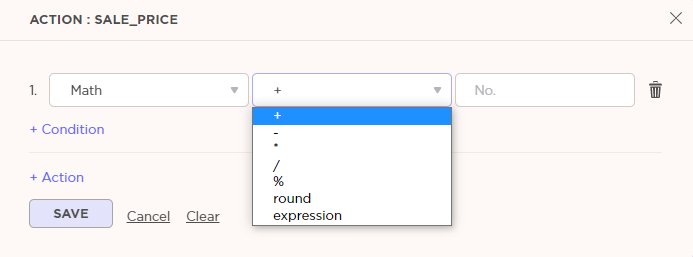Expression

Expression allows us to create calculations across columns or combine values in one column and write any mathematical equations of our choosing.

To write an expression:

• Go to Math.
• Choose “Expression”.
• Enter the mathematical expression you want to have – when choosing a column to take information from, surround the column name with curly brackets {___}.
• Make sure to write the column name exactly like in your feed heading (to have the exact name, choose “Copy Script Column Name” from the drop-down menu, and paste it into the brackets).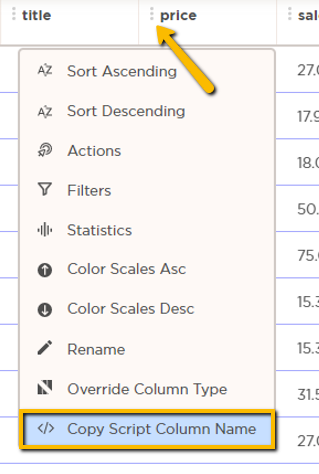Example: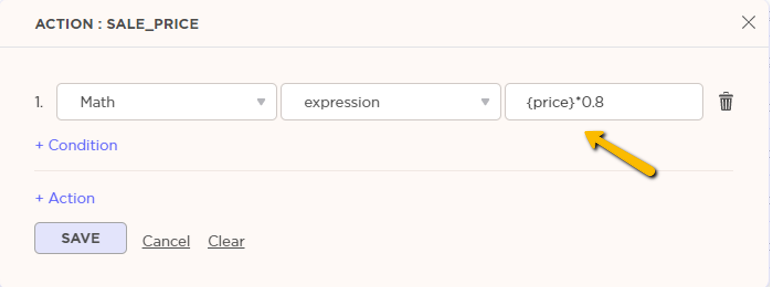NOTE: If you change the field name, use the ORIGINAL FIELD NAME before the change in the expression.

Round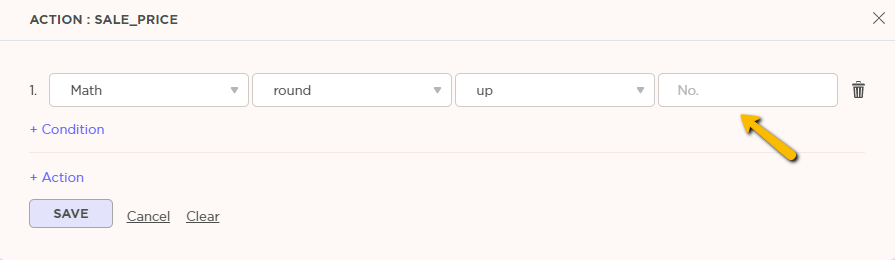This function allows you to round up numbers.

To round up the entire numbers to the nearest round number, choose “0.5”: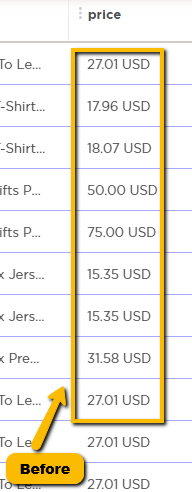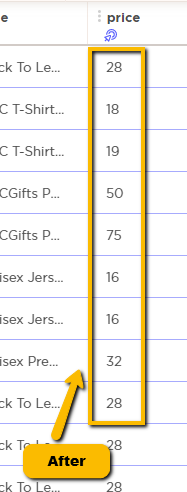To round up the first number that comes after the decimal point, choose “1”: